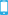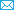•001-646-781-7506
•info@3ge-learning.com

Economics# 3GE Collection on Economics: Econometrics

• ISBN
• Price
• Publication Year
• Publisher
• Binding
• Description
• Preface
• Provide the reader with a steady conceptual and empirical understanding of basic information-theoretic econometric models and methods.
• The applied econometric methods emphasized in this book are user-friendly and relevant for many areas of contemporary social science.
• The book is also well suitable for self-study and can be recommended to everybody who is in need to quickly acquire the basics of the field.

Econometrics is usually referred to the assimilation of economics, mathematical economics and statistics in order to provide numerical standards to the considerations of economic dealings. It deals with the application of mathematical economic theory and statistical procedures to economic data in order to establish numerical results in the field of economics. Econometrics has to be distinguished from mathematical economics and from statistical economics. It is, however, closely related to both, and utilizes results achieved in these fields. The associations of economic theories are commonly conveyed in mathematical terms and integrated with realistic economics. The econometrics methods are applied to attain the values of factors which are essentially the quantities of mathematical form of the economic phenomenon. The statistical methods which assist in clarifying the economic relationship are used as econometric methods. The econometric phenomenon describes the random behaviour of economic relationships which are usually not focused in economics and mathematical foundations.

It may be noted that the econometric methods are utilized in different areas such as medical sciences, geosciences, agricultural sciences, biological sciences, and others. In other words, when there is a need to evaluate the stochastic relationship in mathematical terms, the econometric methods and tools are beneficial in clarifying the relationships among variables.

Econometrics is becoming a highly developed and highly mathematized array of its own sub disciplines, as it should be, as economies are becoming increasingly complex, and scientific economic analyses require progressively thorough knowledge of solid quantitative methods. This book thus provides recent insight on some key issues in econometric theory and applications. It also deals with current trends in econometric theory. This volume summarizes the economic theory, the econometric methodology and the empirical findings resulting from the new approach to econometric modelling of producer behaviour, including analysis and appreciation of the structural econometric, time series analysis approach to statistical and econometric modeling.

For those who want to read on this applied topic, the empirical applications of the book are a good way to learn as well as an assisting guide to conduct empirical research.